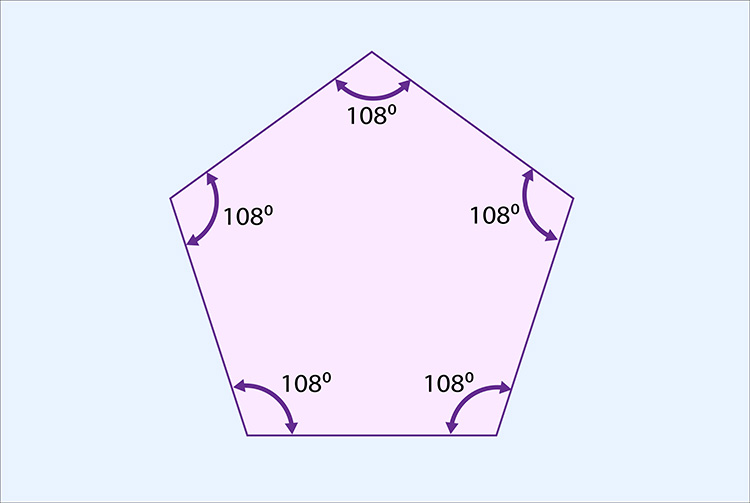# The total interior angles of a pentagon = 540°

Each time we add a side (triangle to square, square to pentagon, pentagon to hexagon) we add another 180°.

## Triangle to pentagon

 Triangle Square Pentagon 180° + 180° + 180° = 540°

The total internal angle of a pentagon = 540°Now divide the total internal angle by the number of corners.

i.e. divide 540° by 5.

540^circ/5=108^circ

 1 2 3 4 5 108° + 108° + 108° + 108° + 108° = 540°